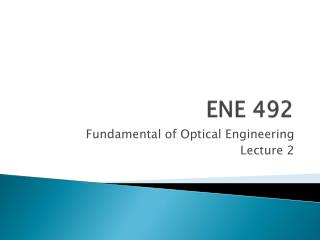# ENE 492 - PowerPoint PPT PresentationDownload PresentationENE 492

ENE 492
Download Presentation## ENE 492

- - - - - - - - - - - - - - - - - - - - - - - - - - - E N D - - - - - - - - - - - - - - - - - - - - - - - - - - -
##### Presentation Transcript

1. ENE 492 Fundamental of Optical Engineering Lecture 2

2. Converging lens • In order to locate the image, the 2 rays are needed as • The parallel ray: parallel to the axis and then, after refraction, passes through another focal point. • The focal ray: passes through a first focal point then, after refraction, is parallel to the axis. • The chief ray goes through the center of the lens without deviation since the lens is thin.

3. Converging lens

4. Derivation of Gauss’ Thin Lens Equation • L.H.S. • R.H.S.

5. Derivation of Gauss’ Thin Lens Equation • Take ratio (1) = (2), we have

6. Derivation of Gauss’ Thin Lens Equation • Multiply both sides by 1/ss’f, this yields • Finally, we have

7. Derivation of Gauss’ Thin Lens Equation • Magnification, • From (2) • From (3) x s’

8. Derivation of Gauss’ Thin Lens Equation • So that,

9. Conventions for lenses • Light travels from left to right. • All distances are measured from the plane of a lens. • Object to the left of a lens is called object distance s, and s is negative. • The image distance s’ could be positive (real image) and negative (virtual image).

10. Conventions for lenses • The focal length, f • f is ‘+’ for converging lens. • f is ‘-’ for diverging lens. • y’ is the height of an image. • y’ is ‘+’ for upright image. • y’ is ‘-’ for inverted image. • Magnification M • M is ‘+’ for upright image. • M is ‘-’ for inverted image.

11. Example • Find s’ and M for these cases of object distances.

12. Off-axis rays • In case of off-axis rays incident on a converging lens, the displacement of spot in focal plane can be found as

13. Off-axis rays • d = ftanθ • If θ is small, then tan θ θ. • d  fθ……Displacement of spot in focal plane.

14. Diverging Lens

15. Diverging Lens • In case of diverging lens, focal length is negative in GTLE. • That leads an image distance to be ‘-’. • This means we always have a virtual image to the left of the lens.

16. Example • For diverging lens, find s’ and M for various s.

17. Lens combinations • We may use the combinations of lenses to form desired images.

18. Example

19. Example

20. Example • When an object is placed 75 mm in front of a converging lens, its image is 3 times as far away from the lens as when the object is at infinity. What is the focal length of the lens?